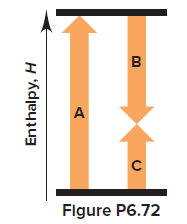# Problem: Write the balanced overall equation (equation 3) for the following process, calculate ΔHoverall, and match the number of each equation with the letter of the appropriate arrow in Figure P6.72:(1) N2 (g) + O2 (g) ⟶ 2NO (g)                Δ  H = 180.6 kJ(2) 2NO (g) + O2 (g) ⟶ 2NO2 (g)           Δ H = −114.2 kJ--------------------------------------------------------------------------------------------(3)                                                 ΔH     overall = ?

⚠️Our tutors found the solution shown to be helpful for the problem you're searching for. We don't have the exact solution yet.

###### Problem Details

Write the balanced overall equation (equation 3) for the following process, calculate ΔHoverall, and match the number of each equation with the letter of the appropriate arrow in Figure P6.72:

(1) N2 (g) + O2 (g) ⟶ 2NO (g)                Δ  H = 180.6 kJ
(2) 2NO (g) + O2 (g) ⟶ 2NO2 (g)           Δ H = −114.2 kJ

--------------------------------------------------------------------------------------------
(3)                                                 ΔH     overall = ?